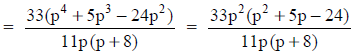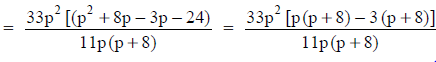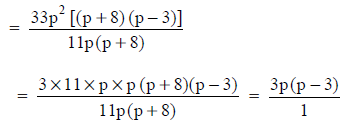Courses

# NCERT Solutions(Part- 2)- Factorisation Class 8 Notes | EduRev

## Class 8 Mathematics by VP Classes

Created by: Full Circle

## Class 8 : NCERT Solutions(Part- 2)- Factorisation Class 8 Notes | EduRev

The document NCERT Solutions(Part- 2)- Factorisation Class 8 Notes | EduRev is a part of the Class 8 Course Class 8 Mathematics by VP Classes.
All you need of Class 8 at this link: Class 8

EXERCISE 14.2
Question 1. Factorise the following expressions.
(i) a2 + 8a + 16
(ii) P2 – 10p + 25
(iii) 25m2 + 30 m + 9
(iv) 49y2 + 84yz + 36z2
(v) 4x2 – 8x + 4
(vi) 121b2 – 88bc + 16c2
(vii) (l + m)2 – 4lm
(viii) a4 + 2a2b2 + b4
[Hint: Expand (l + m)2 first.]

Solution:
(i)
We have   a2 + 8a + 16 = (a)2 + 2(a)(4) + (4)2
= (a + 4)2 = (a + 4)(a + 4)
∴                   a2 + 8a + 16 = (a + 4)2 = (a + 4)(a + 4)

(ii) We have p2 – 10p + 25 = P2 – 2(p)(5) + (5)2
= (p – 5)2 = (p – 5)(p – 5)
∴                  P2 – 10p + 25 = (p – 5)2 = (p – 5)(p – 5)

(iii) We have     25m2 + 30m + 9 = (5m)2 + 2(5m)(3) + (3)2
= (5m + 3)2 = (5m + 3)(5m + 3)
∴                       25m+ 30m + 9 = (5m + 3)2 = (5m + 3)(5m + 3)

(iv) We have 49y2 + 84yz + 36z = (7y)2 + 2(7y)(6z) + (6z)2
= (7y + 6z)2
= (7y + 6z)(7y + 6z)
∴
49y2 + 84yx + 36 z2 = (7y + 6z)2 = (7y + 6z)(7y + 6z)

(v) We have 4x– 8x + 4 = (2x)2 – 2(2x)(2) + (2)2
= (2x – 2)2 = (2x – 2)(2x – 2)
= 2(x – 1)2(x – 1)
= 4(x – 1)(x – 1)
∴                 4x– 8x + 4 = 4(x – 1)(x – 1) = (4(x – 1)2

(vi) We have 121b2 – 88bc + 16c= (11b)2 – 2(11b)(4c) + (4c)2
= (11b – 4c)2 = (11b – 4c)(11b – 4c)
∴                  121b2 – 88bc + 16c2 = (11b – 4c)2 = (11b – 4c)(11b – 4c)

(vii) We have   (l + m)2 – 4lm = (l2 + 2lm + m2) – 4lm
[Collecting the like terms 2lm and –4lm]
= l2 + (2lm – 4lm) + m2
= l2 + 2lm + m2
= (l)2 – 2(l)(m) + (m)2
= (l – m)2 = (l – m)(l – m)
∴                  (l + m)2 – 4lm = (l – m)2 = (l – m)(l – m)

(viii) We have a4 + 2a2b2 + b4 = (a2)2 + 2(a2)(b2) + (b2)2
= (a2 + b2)2 = (a+ b2)(a2 + b2)
∴                   a4 + 2a2b2 + b2 = (a2 + b2)2 = (a2 + b2)(a2 + b2)

Question 2. Factorise:
(i) 4p2 – 9q2
(ii) 63a2 – 112b2
(iii) 49x2 – 36
(iv) 16x5 – 144x3
(v) (l + m)– (l – m)2
(vi) 9x2y2 – 16
(vii) (x2 – 2xy + y2) – z2
(viii) 25a2 – 4b2 + 28bc – 49c2

Solution:
(i)
∵ 4p2 – 9q2 = (2p)2 – (3q)2
= (2p – 3q)(2p + 3q)        [Using a– b2 = (a + b)(a – b)]
∴    4p– 9q2 = (2p – 3q)(2p + 3q)

(ii) We have
63a2 – 112b2 = 7 * 9a2 – 7 * 16b2
= 7[9a2 – 16b2)
∵       9a2 – 16b2 = (3a)2 – (4b)2
= (3a + 4b)(3a – 4b)          [Using a2 – b2 = (a + b)(a – b)]
∴ 63a– 112b2 = 7[(3a + 4b)(3a – 4b)]

(iii) ∵  49 x2 – 36 = (7x)2 – (6)2
= (7x – 6)(7x + 6)              [Using a2 – b2 = (a – b)(a + b)]
∴        49x2 – 36 = (7x – 6)(7x + 6)

(iv) We have 16x= 16x3 * x2 and 144x3 = 16x3 * 9
∴     16x5 – 144x3 = (16x3) * x2 – (16x3) * 9
= 16x3[x2 – 9]
= 16x3[(x)2 – (3)2]
= 16x3[(x + 3)(x – 3)]          [(Using a2 – b2 = (a – b)(a + b)]
∴     16x5 – 144x3 = 16x3(x + 3)(x – 3)

(v) Using the identitiy a2 – b2 = (a + b)(a – b), we have
(l + m)2 – (l – m)2 = [(l + m) + (l – m)][(l + m) – (l – m)]
= [l + m + l – m][l + m – l + m]
= (2l)(2m) = 2 * 2(l * m)
= 4lm

(vi) We have    9x2y2 – 16 = (3xy)2 – (4)2
= (3xy + 4)(3xy – 4)        [Using a2 – b2 = (a + b)(a – b)]
∴                      9x2y2 – 16 = (3xy + 4)(3xy – 4)

(vii) We have x2 – 2xy + y2= (x – y)2
∴         (x2 – 2xy + y2) – z2 = (x – y)2 – (z)2
= [(x – y) + z][(x – y) – z]
[Using a– b2 = (a + b)(a – b)]
= (x – y + z)(x – y – z)
∴       (x2 – 2xy + y2) – z2 = (x – y + z)(x – y – z)

(viii) We have       –4b2 + 28bc – 49c2 = (–1)[4b2 – 28bc + 49c2]
= –1[(2b)– 2(2b)(7c) + (7c)2]
= –[2b – 7c]2
∴                25a2 – 4b2 + 28bc – 49c2  = 25a2 – (2b – 7c)2
Now, using                              a2 – b2 = (a + b)(a – b), we have
[5a]2 – [2b – 7c]2 = (5a + 2b – 7c)(5a – 2b + 7c)
∴               25a2 – 4a2 + 28bc – 49c2 = (5a + 2b – 7c)(5a – 2b + 7c)

Question 3. Factorise the expressions.
(i) ax2 + bx
(ii) 7p2 + 21q2
(iii) 2x3 + 2xy2 + 2xz2
(iv) am2 + bm2 + bn2 + an2
(v) (lm + l) + m + 1
(vi) y(y + z) + 9(y + z)
(vii) 5y2 – 20y – 8z + 2yz
(viii) 10ab + 4a + 5b + 2
(ix) 6xy – 4y + 6 – 9x

Solution:
(i) ∵       ax2 = a * x * x = (x)[ax]
bx = b * x = (x)[b]
∴   ax2 + bx = x[ax + b]

(ii) We have 7p2 + 21q2 = 7 * p * p + 7 * 3 * q * q
= 7[p * p + 3 * q * q]
= 7[p2 + 3q2]

(iii) Taking out 2x as common from each term, we have
2x+ 2xy+ 2xz= 2x[x2 + y2 + z2]

(iv) We can take out m2 as common from the first two terms and n2 as common from the last two terms,
∴ am2 + bm2 + bn2 + an2 = m2(a + b) + n2(b + a)
= m2(a + b) + n2(a + b)
= (a + b)[m2 + n2]

(v) We have     lm + l = l(m + 1)
∴    (lm + l) + (m + 1) = l(m + 1) + (m + 1)
= (m + 1)[l + 1]

(vi) We have (y + z) as a common factor to both terms.
∴     y(y + z) + 9(y + z) = (y + z)(y + 9)

(vii) We have 5y2 – 20y = 5y(y – 4)
and                –8z + 2yz = 2z(–4 + y)
= 2z(y – 4)
∴ 5y2 – 20y – 8z + 2yz = 5y(y – 4) + 2z(y – 4)
= (y – 4)[5y + 2z]

(viii) We have,    10ab + 4a = 2a(5b + 2)
and                         (5b + 2) = 1(5b + 2)
∴           10ab + 4a + 5b + 2 = 2a(5b + 2) + 1(5b + 2)
= (5b + 2)[2a + 1]

(ix) Regrouping the terms, we have
6xy – 4y + 6 – 9x = 6xy – 4y – 9x + 6
= 2y[3x – 2] – 3[3x – 2]
= (3x – 2)[2y – 3]

Question 4. Factorise:
(i) a4 – b4
(ii) p4 – 81
(iii) x4 – (y + z)4
(iv) x4 – (x – z)4
(v) a4 – 2a2b2 + b4

Solution:
(i) Using a2 – b2 = (a – b)(a + b), we have
a4 – b4 = (a2)2 – (b2)2
= (a2 + b2)(a2 – b2)
= (a2 + b2)[(a + b)(a – b)]
= (a2 + b2)(a + b)(a – b)

(ii) We have    P4 – 81 = (p2)2 – (92)2
Now using      a2 – b2 = (a + b)(a – b), we have
(p2)– (9)2 = (p2 + 9)(p2 – 9)
We can factorise p2 – 9 further as
p2 – 9 = (p)– (3)2
= (p + 3)(p – 3)
∴                  p4 – 81 = (p + 3)(p – 3)(p2 + 9)

(iii)  ∵ x4 – (y + z)4 = [x2]2 – [(y + z)2]2
= [(x)2 + (y + z)2][(x2) – (y + z)2]
[Using a2 – b2 = (a + b)(a – b)]
We can factorise [x2 – (y + z)2] further as
(x)2 – (y + z)2 = [(x) + (y + z)][(x) – (y + z)]
= (x + y + z)(x – y – z)
∴       x4 – (y + z)4 = (x + y + z)(x – y – z)[x2 + (y + z)2]

(iv) We have x4 – (x – z)4 = [x2]2 – [(x – z)2]2
= [x2 + (x – z)2][x2 – (x – z)2]
Now, factorising x2 – (x – z)2 further, we have
x2 – (x – z)2 = [x + (x – z)][x – (x – z)]
= (x + x – z)(x – x + z) = (2x – z)(z)
∴                x4 – (y – z)4 = z(2x – z)[x2 + (x – z)2]
= z(2x – z)[x2 + (x2 – 2xz + z2)]
= z(2x – z)[x2 + x2 – 2xz + z2]
= z(2x – z)(2x2 – 2xz + z2)

(v) ∵   a4 – 2a2b2 + b4 = (a2)2 – 2(a2)(b2) + (b2)2
= (a2 – b2)2
= [(a2 – b2)(a2 + b2)]
= [(a – b)(a + b)(a2 + b2)]
∴         a4 – 2a2b+ b= (a – b)(a + b)(a2 + b2)

Question 5. Factorise the following expressions.
(i) p+ 6p + 8
(ii) q2 – 10q + 21
(iii) p2 + 6p – 16

Solution:
(i) We have     p2 + 6p + 8 = P2 + 6p + 9 – 1                [∵ 8 = 9 – 1]
= [(p)+ 2(p)(3) + 32] – 1
= (p + 3)2 – 12      [Using a2 + 2ab + b2 = (a + b)2]
= [(p + 3) + 1][(p + 3) – 1]
= (p + 4)(p + 2)
∴                     p2 + 6p + 8 = (p + 4)(p + 2)

(ii) We have     q– 10q + 21 = q2 – 10q + 25 – 4       [∵ 21 = 25 – 4]
= [(q)2 – 2(q)(5) + (5)2] – (2)2
= [q – 5]2 – 2
= [(q – 5) + 2][(q – 5) – 2]
[Using a2 – b2 = (a + b) (a – b)]
= (q – 3)(q – 7)

(iii) We have      p2 + 6p – 16 = p2 + 6p + 9 – 25          [∵ –16 = 9 – 25]
= [(p)2 + 2(p)(3) + (3)2] – (5)2
= [p + 3]2 – 2 [Using a2 + 2ab + b2 = (a + b)2]
= [p + 3] + 5][(p + 3) – 5]
[Using a2 – b2 = (a + b)(a – b)
= (p + 8)(p – 2)

DIVISION OF ALGEBRAIC EXPRESSIONS
For division of algebraic expression by another expression:
I. We write them in the form of a fraction, such that the divisor is the denominator.
II. Factorise the numerator as well as the denominator.
III. Cancel the factors common to both the numerator and denominator.

Example 1. Divide 33(p4 + 5p3 – 24p2) by 11p(p + 8).
Solution: We have 33(p4 + 5p3 – 24p2) ÷ 11p(p + 8)[In numerator, p is taken out common from each term][In numerator, 5p is splitted in to 8p – 3p such that (8p)(–3p) = –24p][Cancelling the factors 11p and x + 8 common to both the numerator and denominator]
Thus, 33(p4 + 5p3 – 24p2) ÷ 11p(p + 8)
= 3p(p – 3)

Offer running on EduRev: Apply code STAYHOME200 to get INR 200 off on our premium plan EduRev Infinity!

93 docs|16 tests

,

,

,

,

,

,

,

,

,

,

,

,

,

,

,

,

,

,

,

,

,

;